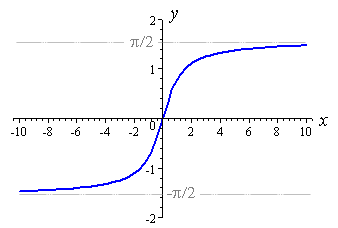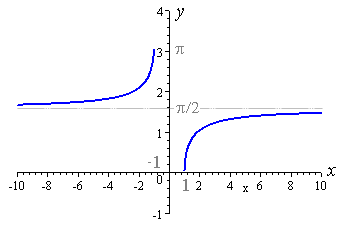# Trig Properties

 tan 1/cot or sin/cos sec 1/cos csc 1/sin cot cos/sin tan, sin, csc, cot Odd trig finction with pi(k), cos, sec Even trig functions with 2(pi)(k) Graph: sin Domain: all real numbers Range: -1, 1 (closed)Graph: cos Domain: all real numbers Range: -1,1 (closed)Graph: csc Domain: all real numbers, expect that theta = (pi)k; k any integer Range: -infinity (open) to -1 (close) Union 1 (closed) to +infinity (open)Graph: sec Domain: all real numbers, expect that theta = (pi/2)k; k any odd integer Range: -infinity (open) to -1 (close) Union 1 (closed) to +infinity (open)Graph: tan Domain: all real numbers, expect that theta = (pi/2)k; k any odd integer Range: all real numbersGaph: cot Domain: all real numbers, expect that theta = (pi)k; k an integer Range: all real numbersGraph: sin-1 Domain: [-1 , 1] Range: [-pi/2 , pi2]Graph: cos-1 Domain: [-1 , 1] Range: [0 , pi]Graph: tan-1 Domain: all real numbers Range: ( -pi/2 , pi/2)Graph: cot-1 Domain: all real numbers Range: (0 , pi)Graph: sec-1 Domain: (-infinity , -1] U [1 , +infinity) Range: [0 , pi] NOT including pi/2Graph: csc-1 Domain: (-infinity , -1] U [1 , +infinity] Range: [-pi/2 , pi/2] NOT including 0Double Angle Foumula: sin(2x) = 2sin(x)cos(x) Double Angle Formula: cos2(x) - sin2(x) 2cos2(x) - 1 Derivative: sin(x) cos(x) Derivative: cos(x) -sin(x) Derivative: tan(x) sec2(x) Derivative: cot(x) -csc2(x) Derivative: sec(x) sec(x)tan(x) Derivative: csc(x) -csc(x)cot(x) Double Angle Formula: cos(2x) 1-2sin2(x) Authorffarouk ID41744 Card SetTrig Properties DescriptionTirg Properties Updated2010-10-17T20:54:30Z Show Answers